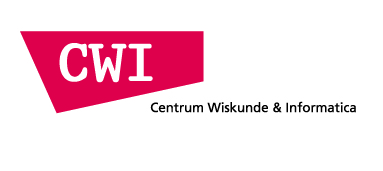CWI Distinguished Scientist Symposium: Quantum information

We are happy to invite you to a symposium with Richard Cleve, who is visiting the Algorithms and Complexity group of CWI as Distinguished Scientist this term. It will take place on
• Thursday July 21, 2011
14:00-17:15
Room L0.17, at the ground floor of the new wing of
CWI. Science Park 123, 1098 XG Amsterdam.
Directions to CWI
The programme consists of four talks, followed by drinks (there is no need to register).

Schedule

• 14:00-14:45 Richard Cleve (Waterloo): Nonlocality in an information processing context
• 14:45-15:30 Serge Massar (Brussels): The certitude of quantum randomness
• Coffee/tea break
• 15:45-16:30 Monique Laurent (CWI and Tilburg): Optimization over polynomials with sums of squares and moment matrices
• 16:30-17:15 Mario Szegedy (Rutgers): Quantum query complexity of state conversion
• Drinks

Abstracts

• 14:00-14:45 Richard Cleve (Waterloo): Nonlocality in an information processing context

Abstract: Nonlocality refers to quantum mechanical effects where two (or more) physically-separated systems can exhibit correlations that are impossible to attain in classical physics. These effects were first studied almost fifty years ago as a means of understanding quantum mechanical behavior. However, in more recent decades, information-processing concepts related to distributed computing have emerged that have put non-locality in a new light. The interplay between physics and computing has led to some fruitful results in both fields. I will survey a few of the key results in this area.

• 14:45-15:30 Serge Massar (Brussels): The certitude of quantum randomness

Abstract: Randomness is a fascinating topic. Its very existence is questioned by philosophers. On the other hand it is very widely used in our information based society. So what is the best approach for generating random numbers? Quantum mechanics, the theory of microscopic particles, offers solutions. In particular by using two entangled particles and the non local correlations they can exhibit, one can generate random numbers with security and reliability impossible with any other scheme. We will present this approach; and also discuss the quantitative relationship between the degree of entanglement, of non locality, and of randomness that can be certified in this way.

• 15:45-16:30 Monique Laurent (CWI and Tilburg): Optimization over polynomials with sums of squares and moment matrices

Abstract: Polynomial optimization deals with the problem of minimizing a multivariate polynomial over a basic closed semi-algebraic set K defined by polynomial inequalities and equations. While polynomial time solvable when all polynomials are linear (via linear programming), the problem is hard in general as soon as it involves non-linear polynomials. A natural approach is to relax the problem and to consider easier to solve, convex relaxations. The basic idea, which goes back to work of Hilbert, is to relax non-negative polynomials by sums of squares of polynomials, a notion which can be tested efficiently (using semidefinite programming). On the dual side, one views points as (atomic) measures and one searches for efficient conditions characterizing sequences of moments of non-negative measures on the semi-algebraic set K. In this way hierarchies of efficient convex relaxations can be build. We will review their various properties. In particular, convergence properties, that rely on real algebraic geometry representation results for positive polynomials, stopping criteria and extraction of global minimizers, that rely on results from moment theory and commutative algebra. We will mention how the methodology extends to polynomial optimization problems with non-commutative variables.

• 16:30-17:15 Mario Szegedy (Rutgers): Quantum query complexity of state conversion

Abstract: State-conversion generalizes query complexity to the problem of converting between two input-dependent quantum states by making queries to the input. We characterize the complexity of this problem by introducing a natural information-theoretic norm that extends the Schur product operator norm. The complexity of converting between two systems of states is given by the distance between them, as measured by this norm. In the special case of function evaluation, the norm is closely related to the general adversary bound, a semi-definite program that lower-bounds the number of input queries needed by a quantum algorithm to evaluate a function. We thus obtain that the general adversary bound characterizes the quantum query complexity of any function whatsoever. This generalizes and simplifies the proof of the same result in the case of boolean input and output. Also in the case of function evaluation, we show that our norm satisfies a remarkable composition property, implying that the quantum query complexity of the composition of two functions is at most the product of the query complexities of the functions, up to a constant. Finally, our result implies that discrete and continuous-time query models are equivalent in the bounded-error setting, even for the general state-conversion problem.
Joint work with Troy Lee, Rajat Mittal, Ben W. Reichardt and Robert Spalek

This page is maintained by Ronald de Wolf. Last update: July 20, 2011